# Linear equation forms questions and answersRecent questions in Forms of linear equations
Forms of linear equations
ANSWERED### The reduced row echelon form of a system of linear equations is given.Write the system of equations corresponding to the given matrix. Use x, y. or x, y, z. or $$x_1, x_2, x_3, x_4$$ as variables. Determine whether the system is consistent or inconsistent. If it is consistent, give the solution. $$\begin{bmatrix}1 & 0 & 0 & 0 & 1\\ 0 &1 & 0 &0 & 2 \\ 0 & 0 & 1 & 2 & 3 \end{bmatrix}$$

Forms of linear equations
ANSWERED### Consider the system (*) whose coefficient matrix A is the matrix D listed in Exercise 46 and whose fundamental matrix was computed just before the preceding exercise.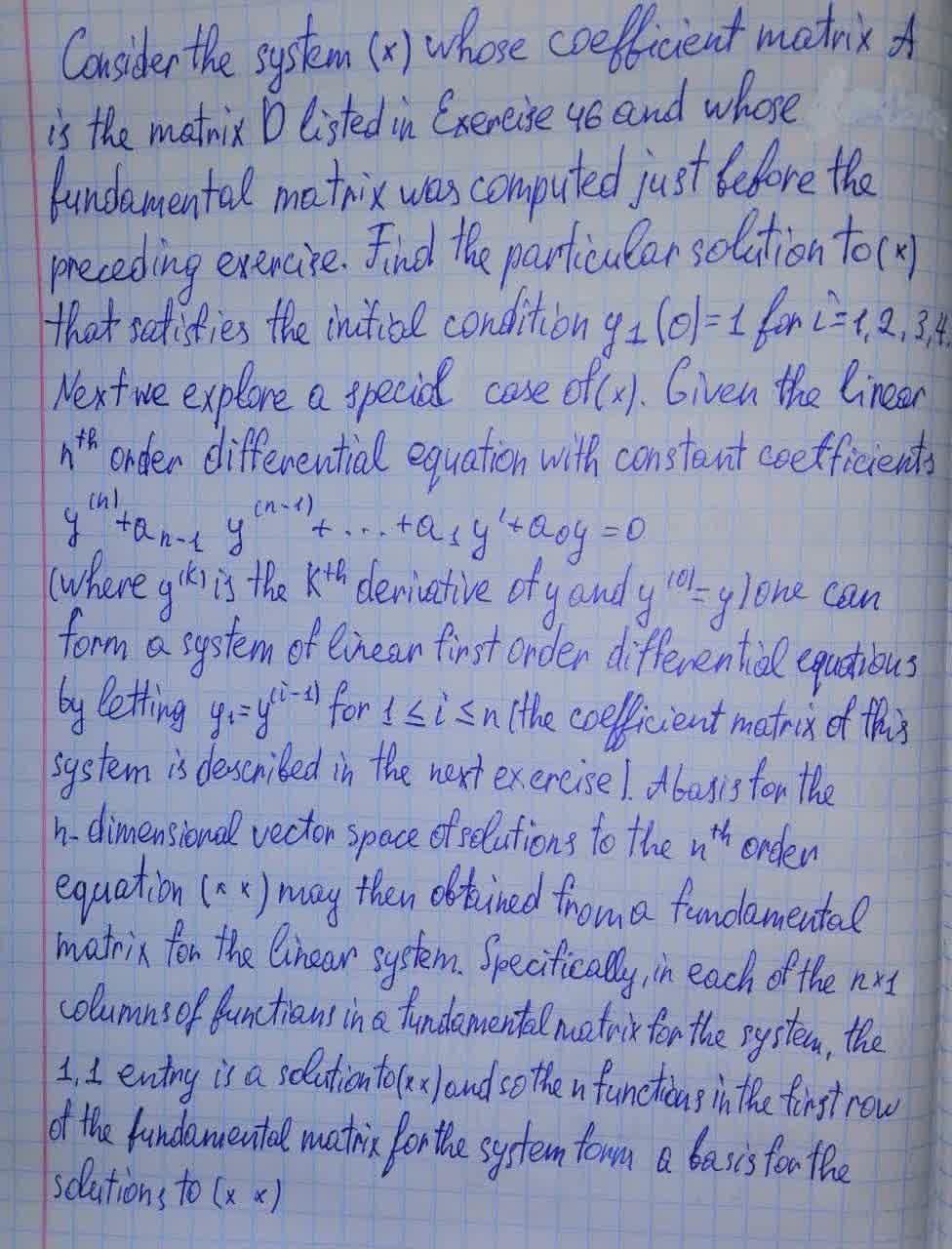Forms of linear equations
ANSWERED### Write the vector form of the general solution of the given system of linear equations. $$\displaystyle-{x}_{{1}}+{2}{x}_{{3}}-{5}{x}_{{4}}+{x}_{{5}}-{x}_{{6}}={0}$$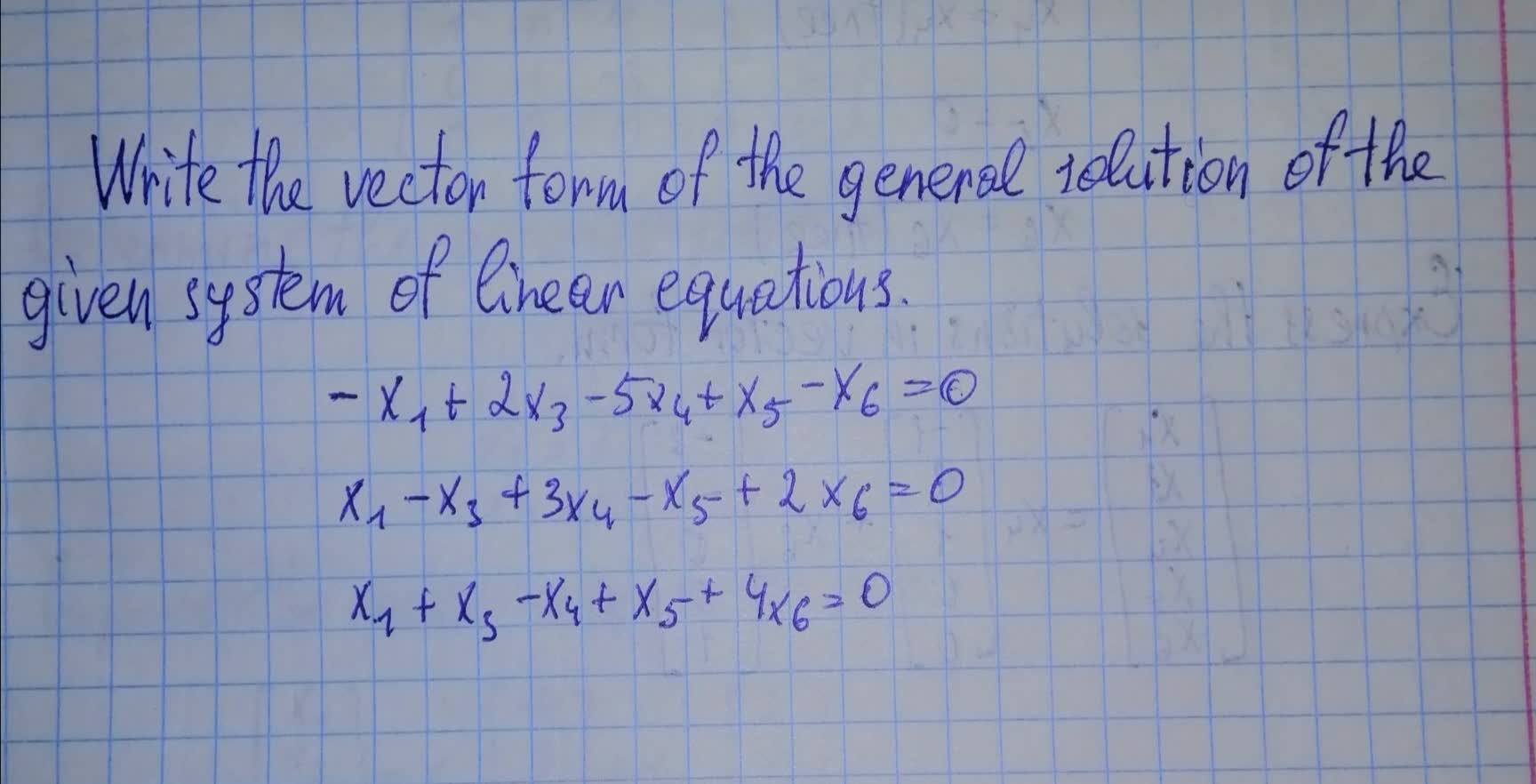Forms of linear equations
ANSWERED### The row echelon form of a system of linear equations is given. a) Write the system of equations corresponding to the given matrix. Use x, y. or x, y, z. or $$x_1,x_2,x_3,x_4$$ as variables. Determine whether the system is consistent or inconsistent. If it is consistent, give the solution. $$\begin{bmatrix}1 & 2 & -1 & 0 \\ 0 & 1 & -1 & 1 \\ 0 & 0 & 0 & 2 \end{bmatrix}$$

Forms of linear equations
ANSWERED### Write the vector form of the general solution of the given system of linear equations. $$\displaystyle{x}_{{1}}+{2}{x}_{{2}}-{x}_{{3}}+{2}{x}_{{5}}-{x}_{{6}}={0}$$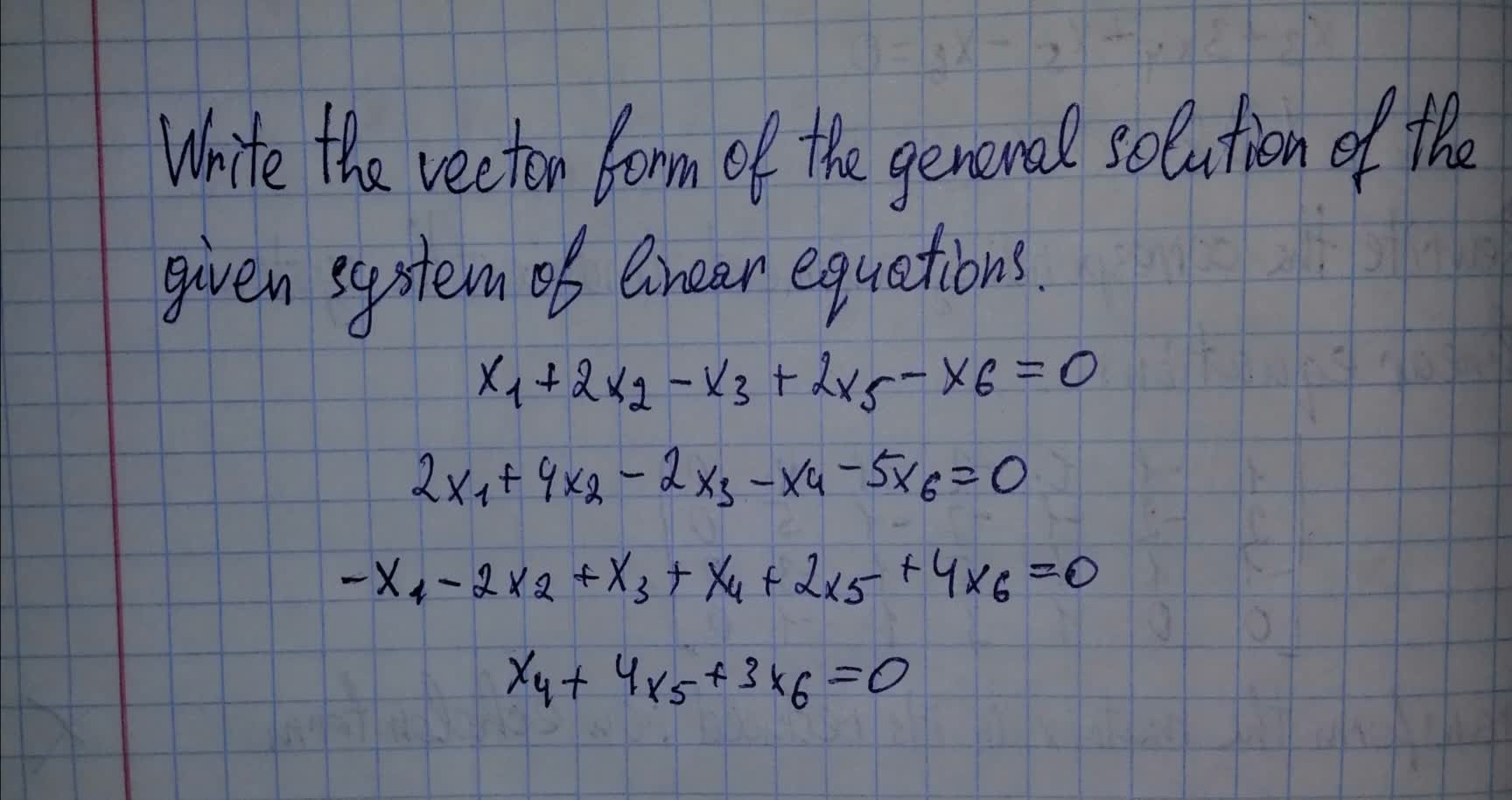Forms of linear equations
ANSWERED### The given matrix is the augmented matrix for a system of linear equations. Give the vector form for the general solution. $$\begin{bmatrix} {1}&{0}&-{1}&-{2}&-{3}&{1}\\{0}&{1}&{2}&{3}&{4}&{0}\end{bmatrix}$$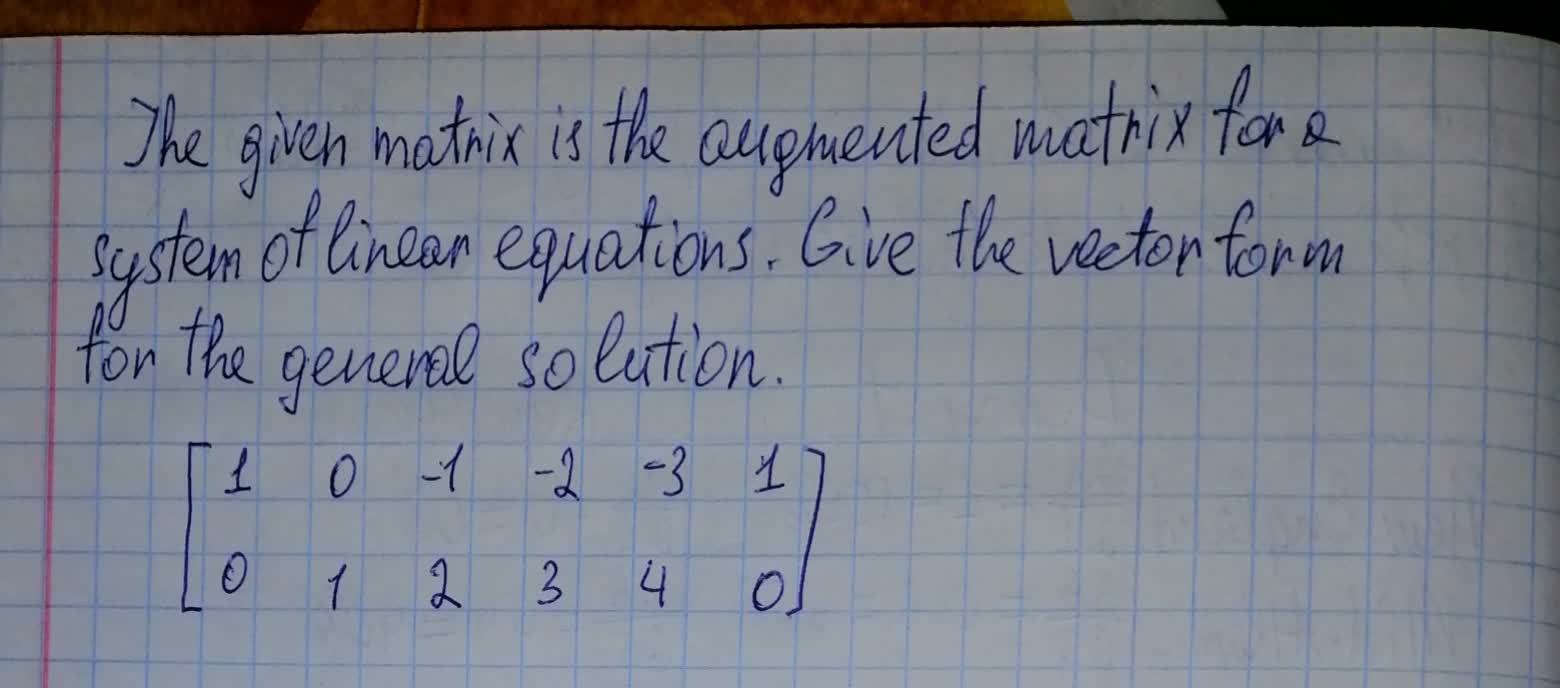Forms of linear equations
ANSWERED### The given matrix is the augmented matrix for a system of linear equations. Give the vector form for the general solution. $$\begin{bmatrix}{1}&{0}&-{1}&-{2}&-{3}&{0}\\{0}&{1}&{2}&{3}&{4}&{0}\end{bmatrix}$$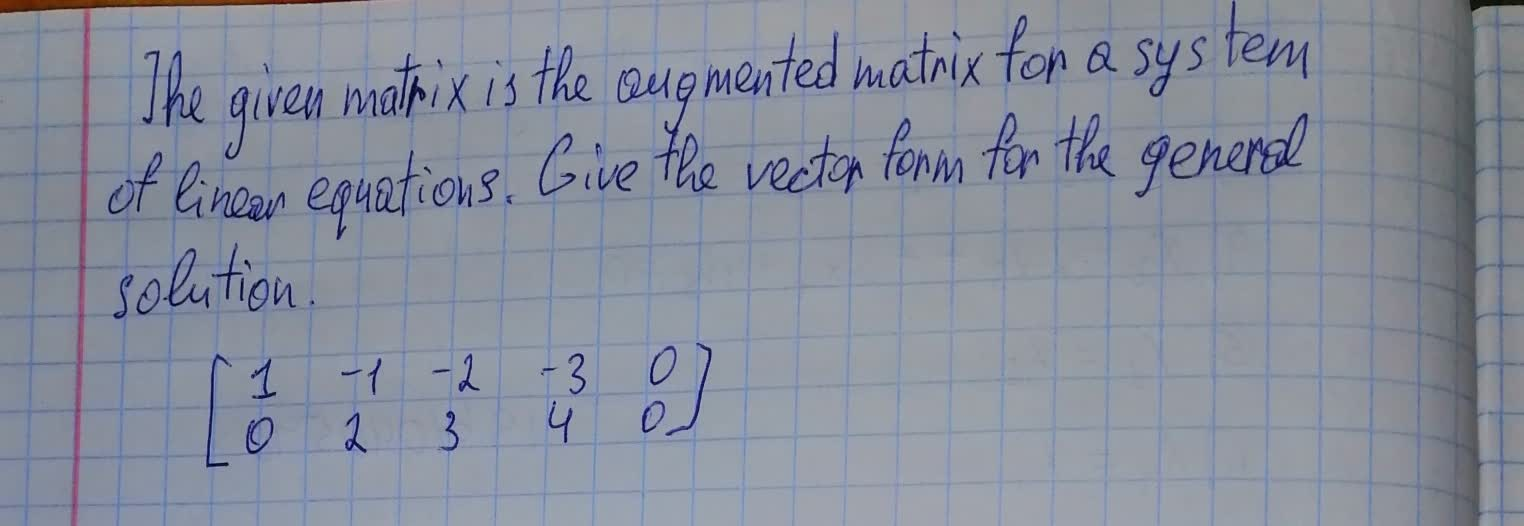Forms of linear equations
ANSWERED### The reduced row echelon form of the augmented matrix of a system of linear equations is given. Determine whether this system of linear equations is consistent and, if so, find its general solution. $$\begin{bmatrix} {0}&{0}&{1}&-{3}&{0}&{2}&{0}\\ {0}&{0}&{0}&{0}&{1}&-{1}&{0}\\ {0}&{0}&{0}&{0}&{0}&{0}&{0}\\ \end{bmatrix}$$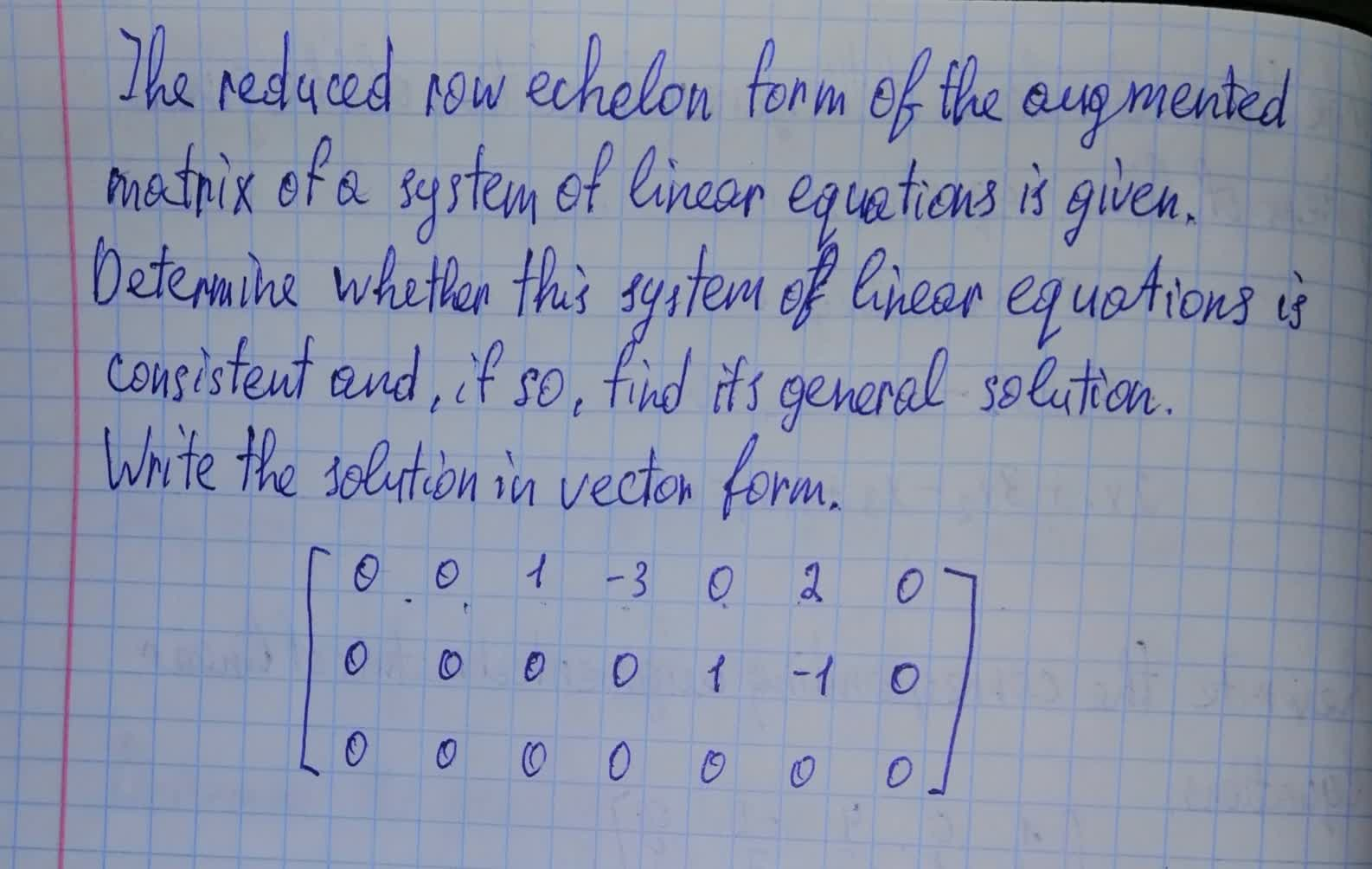Forms of linear equations
ANSWERED### Write the vector form of the general solution of the given system of linear equations. $$\displaystyle{x}_{{1}}+{4}{x}_{{3}}-{2}{x}_{{4}}={0}$$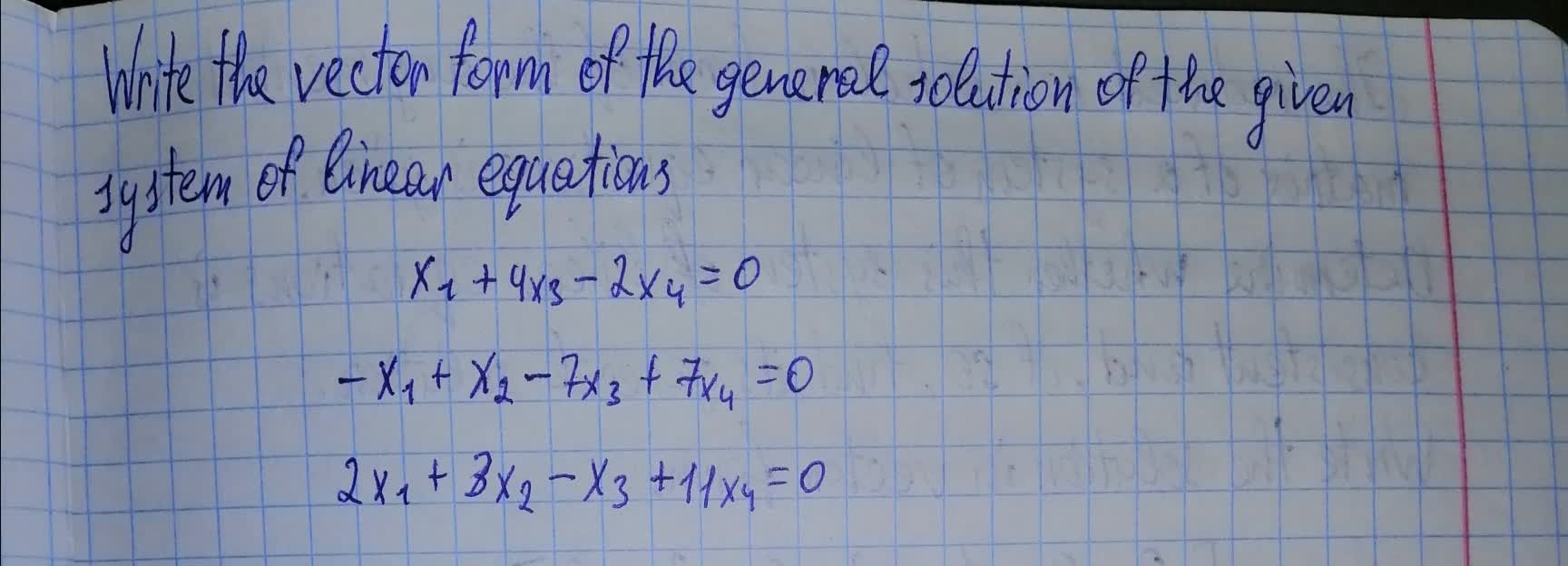Forms of linear equations
ANSWERED### The coefficient matrix for a system of linear differential equations of the form $$\displaystyle{y}^{{1}}={A}_{{y}}$$ has the given eigenvalues and eigenspace bases. Find the general solution for the system.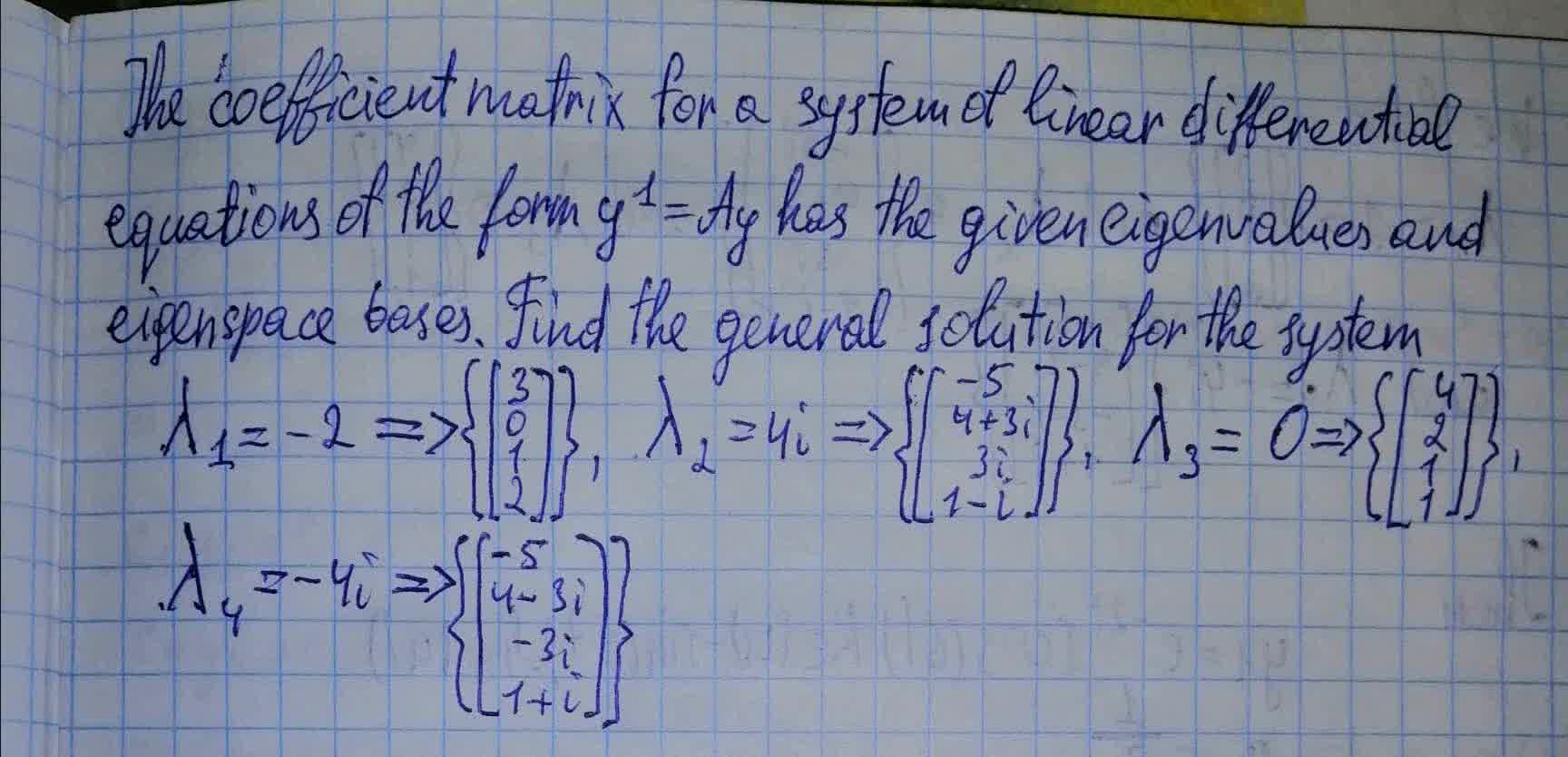Forms of linear equations
ANSWERED### Write the vector form of the general solution of the given system of linear equations. $$\displaystyle{x}_{{1}}-{x}_{{2}}-{2}{x}_{{4}}-{x}_{{5}}+{4}{x}_{{6}}={0}$$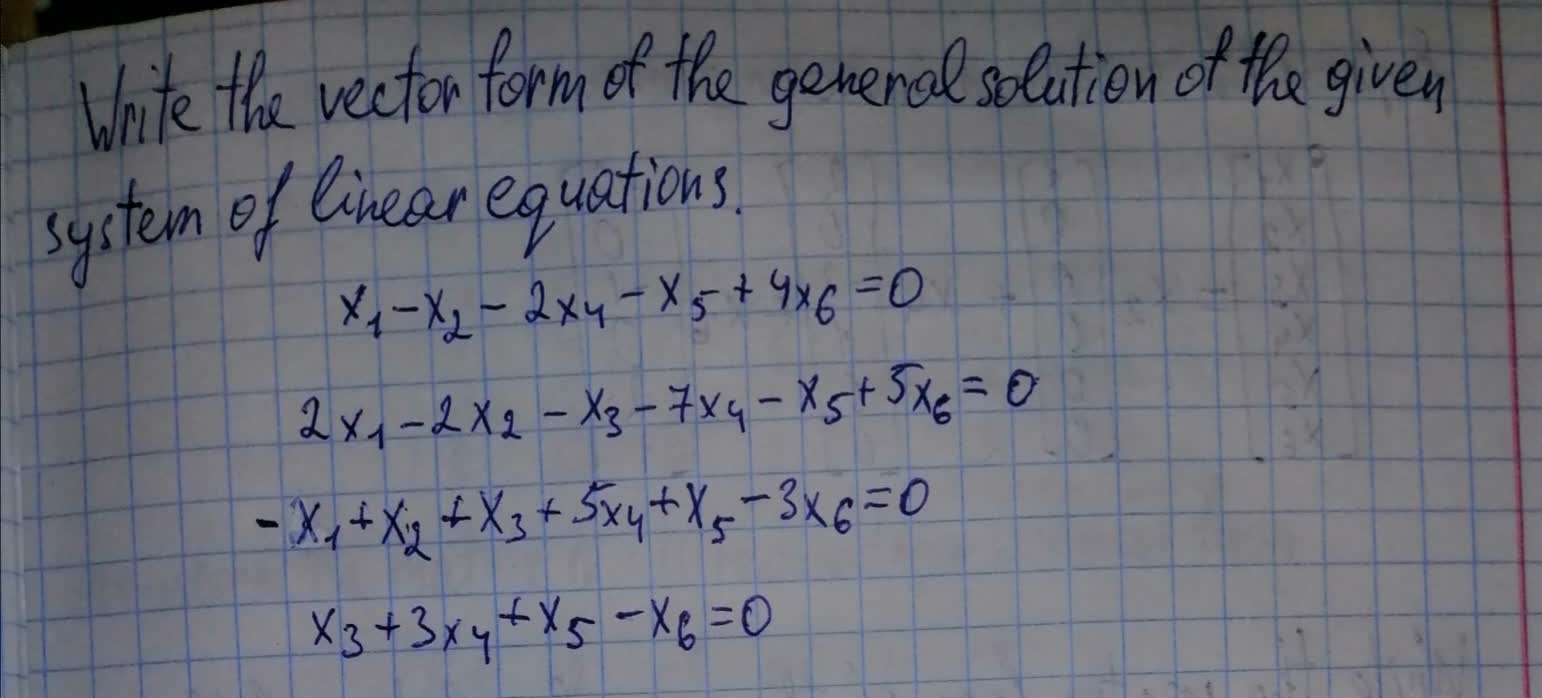Forms of linear equations
ANSWERED### The given matrix is the augmented matrix for a system of linear equations $$\Bigg[ \begin{array}{} 1&0&-1&0&-1&-2&0\\ 0&1&2&0&1&2&0 \\ 0&0&0&1&1&1&0\\ \end{array} \Bigg]$$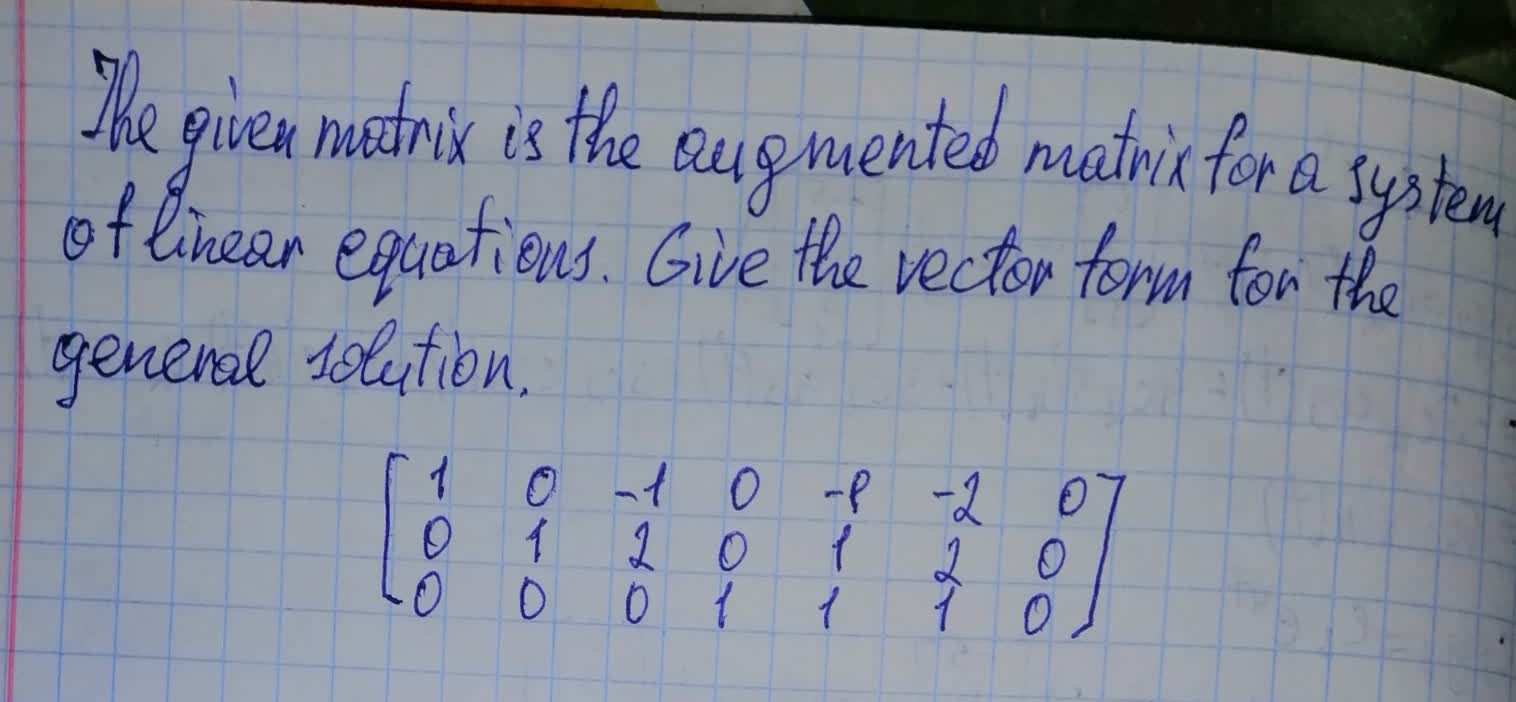Forms of linear equations
ANSWERED### Suppose that the augmented matrix for a system of linear equations has been reduced by row operations to the given row echelon form. Solve the system by back substitution. Assume that the variables are named $$\displaystyle{x}_{{1}},{x}_{{2}},\ldots$$ from left to right. $$\begin{bmatrix}1 & 1&-3&2&1 \\0&1&4&0&3\\0&0&0&1&2 \end{bmatrix}$$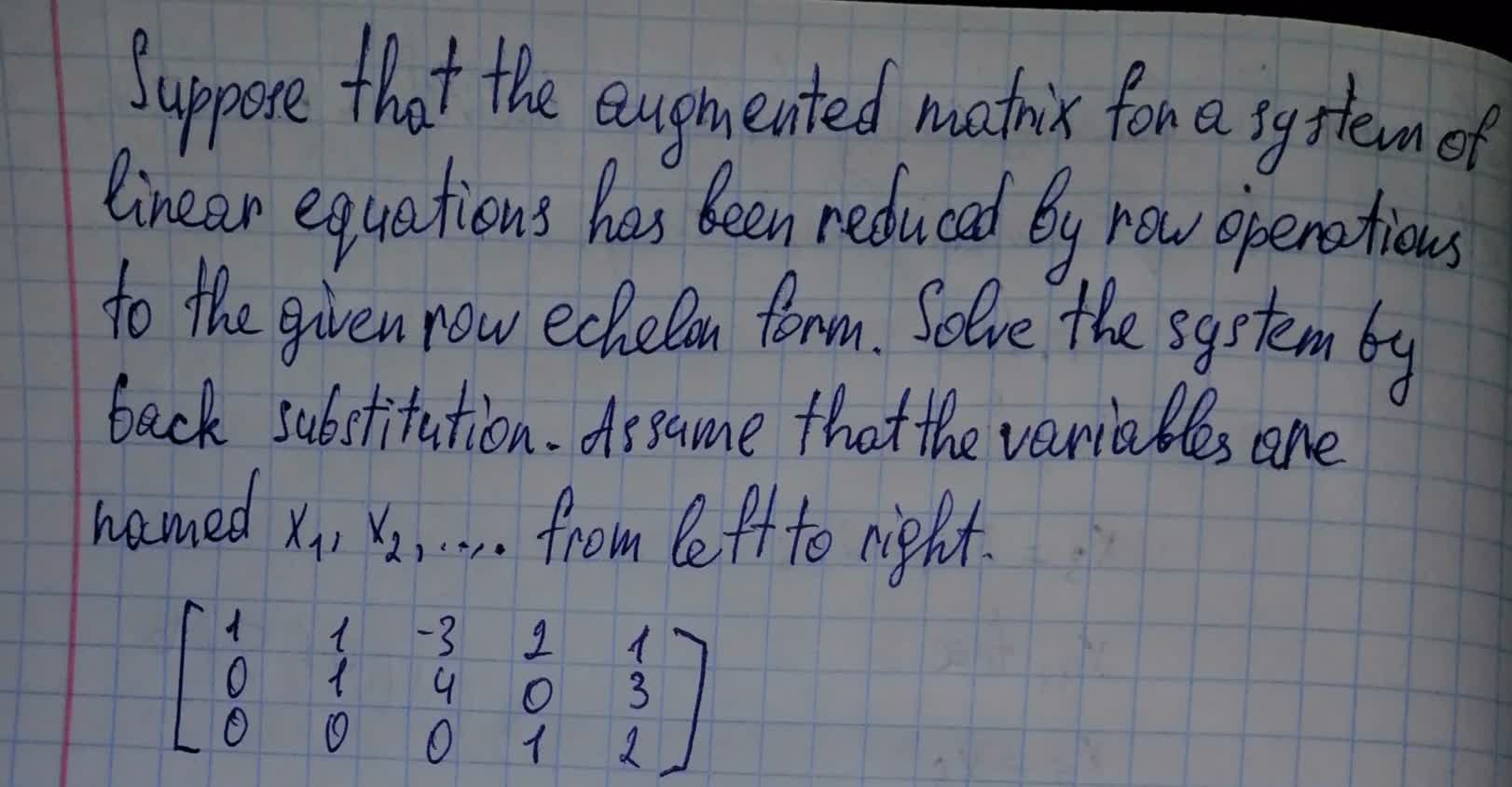Forms of linear equations
ANSWERED### Write the vector form of the general solution of the given system of linear equations. $$\displaystyle{x}_{{1}}-{2}{x}_{{2}}+{x}_{{3}}+{x}_{{4}}+{7}{x}_{{5}}={0}$$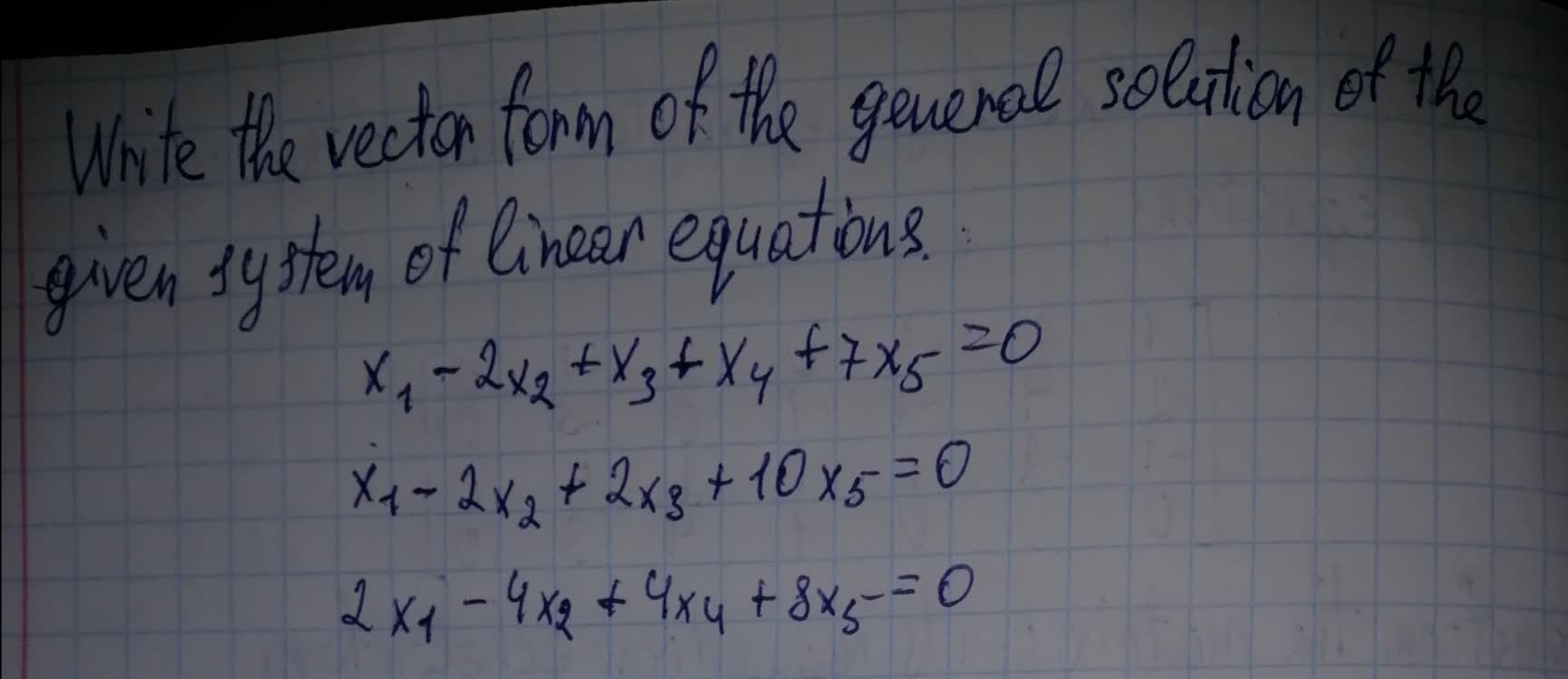Forms of linear equations
ANSWERED### Suppose that the augmented matrix for a system of linear equations has been reduced by row operations to the given reduced row echelon form. Solve the system. Assume that the variables are named $$x_1, x_2,...x,...$$ ,… from left to right.

Forms of linear equations
ANSWERED### Let $$AX = B$$ be a system of linear equations, where A is an $$m\times nm\times n$$ matrix, X is an n-vector, and $$BB$$ is an m-vector. Assume that there is one solution $$X=X0$$. Show that every solution is of the form $$X0+Y$$, where Y is a solution of the homogeneous system $$AY = 0$$, and conversely any vector of the form $$X0+Y$$ is a solution.

Forms of linear equations
ANSWERED### The reduced row echelon form of a system of linear equations is given.Write the system of equations corresponding to the given matrix. Use x, y; or x, y, z; or $$x_1,x_2,x_3,x_4$$ as variables. Determine whether the system is consistent or inconsistent. If it is consistent, give the solution. $$\left[\begin{array}{cccc|c} 1&0&0&4&2\\0&1&1&3&3\\0&0&0&0&0 \end{array}\right]$$

Forms of linear equations
ANSWERED### The reduced row echelon form of a system of linear equations is given.Write the system of equations corresponding to the given matrix. Use x, y; or x, y, z; or $$x_1,x_2,x_3,x_4$$ as variables. Determine whether the system is consistent or inconsistent. If it is consistent, give the solution. $$\left[\begin{array}{ccc|c}{1} & {0} & {4} & {4} \\ {0} & {1} & {3} & {2} \\ {0} & {0} & {0} & {0}\end{array}\right]$$

Forms of linear equations
ANSWEREDANSWERED